Homework Help Question & Answers

# For the reaction 2802(g) + O2(g) -2503(g) AH° = -197.8 kJ and AS° = -187.9 J/K...For the reaction 2802(g) + O2(g) -2503(g) AH° = -197.8 kJ and AS° = -187.9 J/K The equilibrium constant for this reaction at 277.0 K is Assume that AH° and ASº are independent of temperature.

#### Homework Answers

Answer #1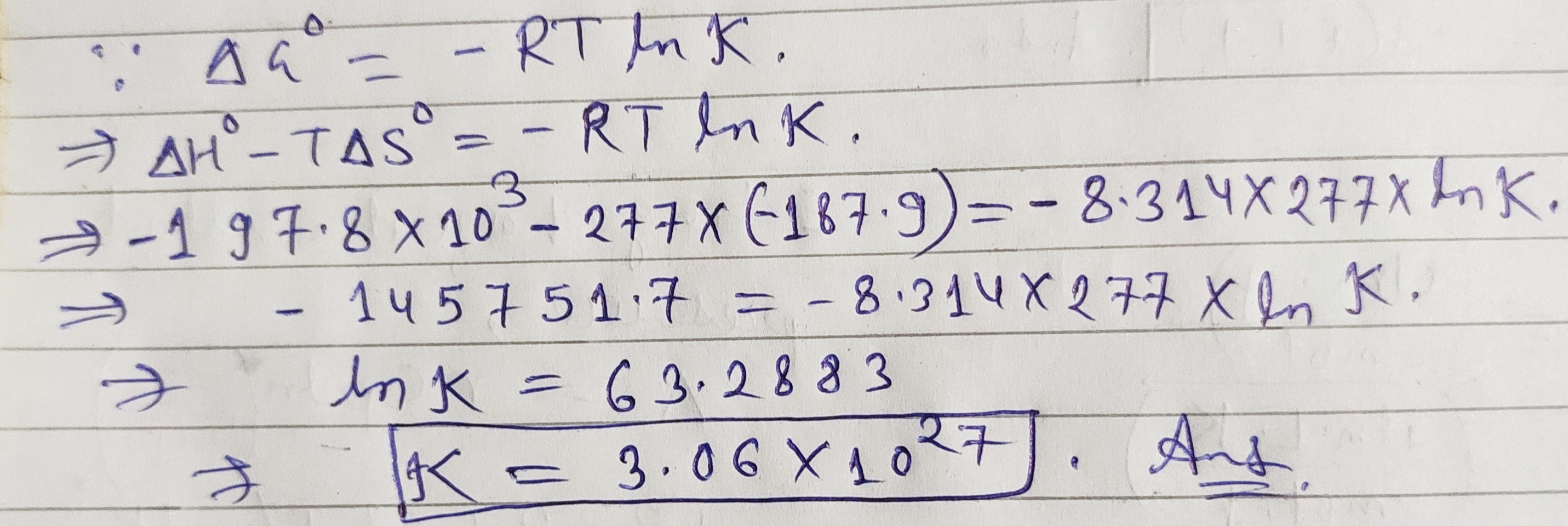Know the answer?
Your Answer:

#### Post as a guest

Your Name:

What's your source?

#### Earn Coin

Coins can be redeemed for fabulous gifts.

Not the answer you're looking for? Ask your own homework help question. Our experts will answer your question WITHIN MINUTES for Free.
Similar Homework Help Questions
• ### For the reaction 2802(g) + O2(g)—>2503(g) AH° = -197.8 kJ and AS° = -187.9 J/K The...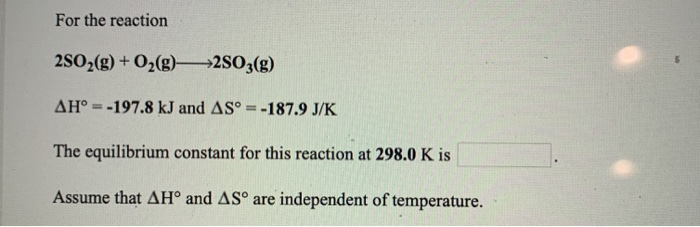For the reaction 2802(g) + O2(g)—>2503(g) AH° = -197.8 kJ and AS° = -187.9 J/K The equilibrium constant for this reaction at 298.0 K is Assume that AHⓇ and AS™ are independent of temperature.

• ### 11. For the reaction Fe2O3(s) + 3H2(g) +2Fe(s) + 3H2O(g) AH° = 98.8 kJ and AS°...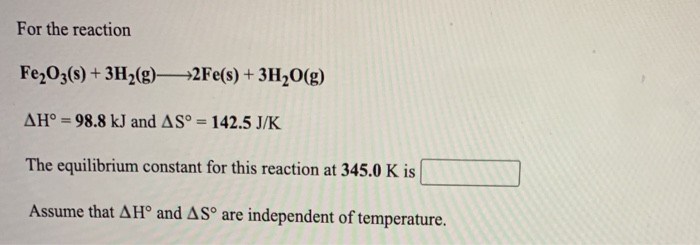11. For the reaction Fe2O3(s) + 3H2(g) +2Fe(s) + 3H2O(g) AH° = 98.8 kJ and AS° = 142.5 J/K The equilibrium constant for this reaction at 345.0 K is Assume that AHⓇ and AS are independent of temperature. For the reaction 2502(g) + O2(g) +2503(g) AH° = -197.8 kJ and AS° = -187.9 J/K The equilibrium constant for this reaction at 272.0 K is Assume that AH° and AS are independent of temperature.

• ### For the reaction N2(g) + O2(g)->2NO(g) AH° = 180.6 kJ and AS = 24.9 J/K The...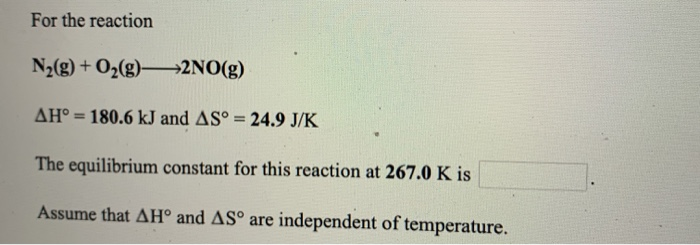For the reaction N2(g) + O2(g)->2NO(g) AH° = 180.6 kJ and AS = 24.9 J/K The equilibrium constant for this reaction at 267.0 K is Assume that AHⓇ and ASº are independent of temperature.

• ### For the reaction N2(g) + O2(g)2NO(g) AH° - 180.6 kJ and AS - 24.9 J/K The...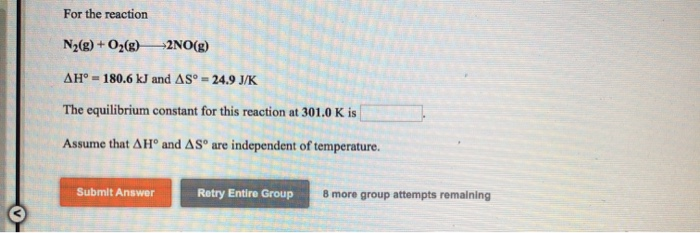For the reaction N2(g) + O2(g)2NO(g) AH° - 180.6 kJ and AS - 24.9 J/K The equilibrium constant for this reaction at 301.0K is Assume that A Hº and AS are independent of temperature. Submit Answer Retry Entire Group 8 more group attempts remaining

• ### For the reaction 2NO(g) + O2(g) —>2NO2(g) AH° = -114.2 kJ and AS° = -146.5 J/K...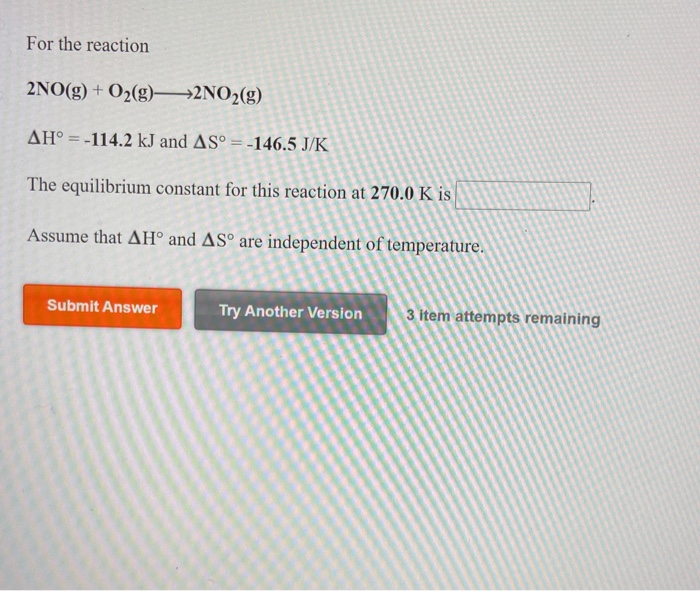For the reaction 2NO(g) + O2(g) —>2NO2(g) AH° = -114.2 kJ and AS° = -146.5 J/K The equilibrium constant for this reaction at 270.0 K is Assume that AH° and AS are independent of temperature. Submit Answer Try Another Version 3 item attempts remaining elefchces to access important values if needed for this question, Consider the reaction 2CO2(g) + 5H2(g)CH2(g) + 4H2O(g) Using the standard thermodynamic data in the tables linked above, calculate AG for this reaction at 298.15K if...

• ### For the reaction 4HCl(g) + O2(g)— *2H2O(g) + 2Cl2(9) AH' = -114.4 kJ and AS° =...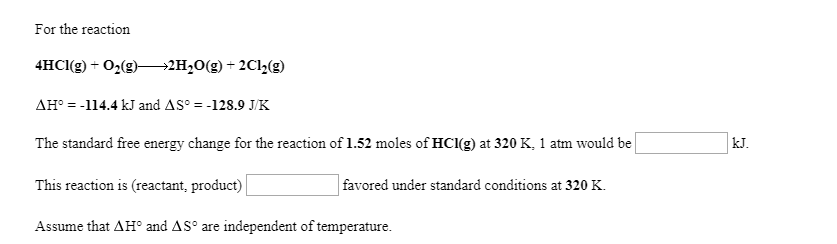For the reaction 4HCl(g) + O2(g)— *2H2O(g) + 2Cl2(9) AH' = -114.4 kJ and AS° = -128.9 J/K The standard free energy change for the reaction of 1.52 moles of HCI(g) at 320 K, 1 atm would be This reaction is (reactant, product) favored under standard conditions at 320 K. Assume that AH and AS are independent of temperature. For the reaction 2 SO2(g) +02(9) —2 SO3(9) AG° = -144.4 kJ and AS° = -187.9 J/K at 284 K and...

• ### For the reaction Fe(s) + 2HCl(aq) FeCl2(5) + H2(g) AH° = -7.4 kJ and AS° =...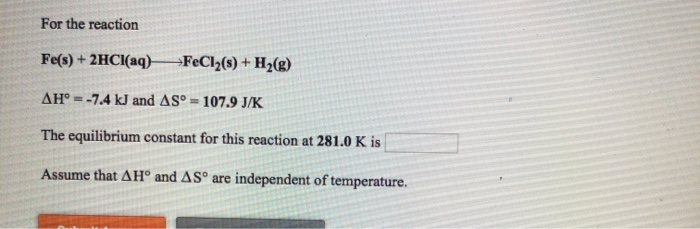For the reaction Fe(s) + 2HCl(aq) FeCl2(5) + H2(g) AH° = -7.4 kJ and AS° = 107.9 J/K The equilibrium constant for this reaction at 281.0 K is Assume that AHº and ASº are independent of temperature.

• ### Given the following data: 3 s(s) + 2O2(9) S03(9) AH = -395.2 kJ 2802(g) + O2(g)...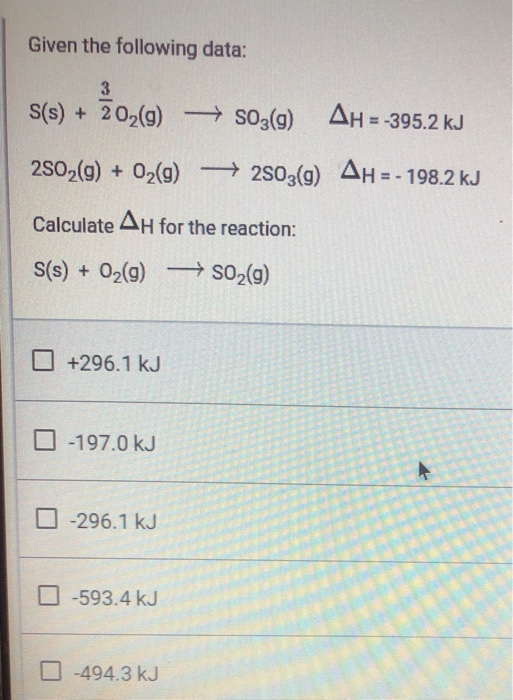Given the following data: 3 s(s) + 2O2(9) S03(9) AH = -395.2 kJ 2802(g) + O2(g) +2503(9) AH = - 198.2 kJ Calculate AH for the reaction: S(s) + O2(g) → SO2(g) +296.1 kJ -197.0 kJ -296.1 kJ -593.4 kJ -494.3 kJ

• ### 2NO(g)02(g)2NO2(g) AH° =-114.2 kJ and AS° =-146.5 J/K The equilibrium constant for this reaction at 321.0...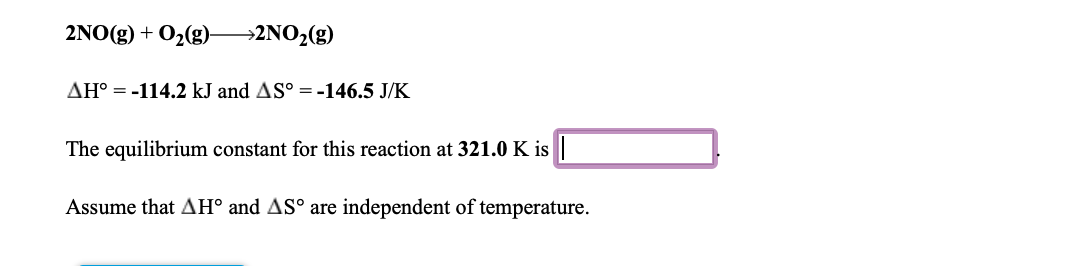2NO(g)02(g)2NO2(g) AH° =-114.2 kJ and AS° =-146.5 J/K The equilibrium constant for this reaction at 321.0 K is Assume that AH° and AS° are independent of temperature

• ### 4. For the reaction Ny() + O2()—>2NO(g) AH-181 kJ and AS - 24.9 J/K AGⓇ would...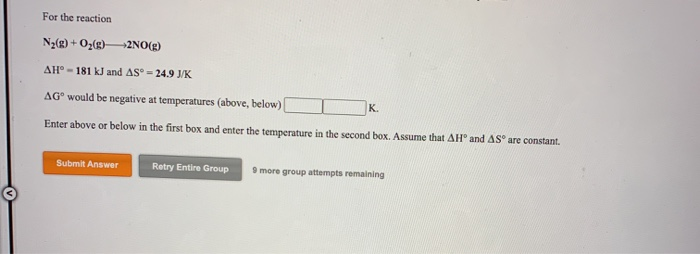4. For the reaction Ny() + O2()—>2NO(g) AH-181 kJ and AS - 24.9 J/K AGⓇ would be negative at temperatures (above, below) Enter above or below in the first box and enter the temperature in the second box. Assume that AHⓇ and AS are constant. K Submit Answer Retry Entire Group 9 more group attempts remaining For the reaction CaCO3(s) CaO(s) + CO2(g) AH° -178 kJ and AS - 161 J/K AGⓇ would be negative at temperatures (above, below) K...

Free Homework App

Scan Your Homework
to Get Instant Free Answers
Need Online Homework Help?

Get Answers For Free
Most questions answered within 3 hours.